Latest Banking jobs   »   Quantitative Aptitude Quiz For SBI Clerk...

# Quantitative Aptitude Quiz For SBI Clerk Prelims 2021- 6th May

Q1. A two digit number when increase by 75% then its digits gets interchanged. If difference between both digits is 3 then find the original number?
(a) 63
(b) 58
(c) 47
(d) 36
(e) Cannot be determined

Q2. Average of Shehwag in 8 innings of first series is 47 runs/innings. In second series of some innings, his average was 3 runs more than the previous average and overall average for both series becomes 48. Find number of innings he played in second series.
(a) 6
(b) 4
(c) 10
(d) 12
(e) None of these

Q3. A positive number is increased by 25%, decrease by 30% and divide by 2. If resultant is multiple by the original number then we get 2800. Find original number.
(a) 40
(b) 70
(c) 60
(d) 100
(e) 80

Q4. To make a toy, 30% raw material cost, 20% worker cost, 30% marketing cost and 20% packing cost occur. If price of raw material is increased by 50% then to get some profit % (20%) price has to increase 450 Rs. Find the cost of packing (in Rs.) ?
(a) 400
(b) 500
(c) 600
(d) 300
(e) 1000

Q5. Sum of a two digit number and reverse of it is 22 times the difference of the digits of the number. Find the two digit number.
(a) 26
(b) 13
(c) 39
(d) all of the above
(e) None

Q6. In an election only two candidates participate. Candidate ‘P’ got 50% less votes than ‘Q’. Had Q got 200 votes less there would have been a tie. What is the 8 times of the number of total votes polled.
(a) 800
(b) 7200
(c) 3200
(d) 9600
(e) 3600

Q7. Average weight of n students in 35. A student whose weight is 25 is absent from the class then average weight of remaining student goes up by 1. Find the value of ‘n’.
(a) 10
(b) 9
(c) 8
(d) 12
(e) 11

Q8. Sumit has some money and want to buy a laptop worth of 35,000. Abhishek gives him 3/2 times of money what Sumit have and Veer contributes 3 times of what Abhishek gives. If he just purchased the laptop then find Abhishek’s contribution.
(a) 7500
(b) 10000
(c) 8000
(d) None of these
(e) 9000

Q9. A number is increased by 350% and divided by half of itself then resultant becomes 45% of itself. What is the original number.
(a) 9
(b) 40
(c) 25
(d) 20
(e) cannot be determined

Q10. If x = |y| – 10, then the minimum possible value of x is.
(a) 0
(b) –10
(c) –5
(d) 10
(e) None of these

Q11. The smallest number of five consecutive odd number series is 3 more than second largest number of five consecutive even number series. Then find average of five consecutive even number series is how much less than that of average of odd number series?
(a) 6
(b) 7
(c) 8
(d) 9
(e) 10

Q12. Arjun can shoot 12 arrows in a minute & Karan can shoot 16 arrows in a minute. If success rate [target killed in 100 arrows] is 60% for Arjun and x% for Karan. If in 5 minutes they both hunt 60 birds, then find value of x.
(a) 60%
(b) 40%
(c) 30%
(d) 36%
(e) 24%

Q13. 20 boys go for dinner. 16 of them spent Rs. 64 each on their dinner and rest spent Rs 4 more than the average expenditure of all 20. What was the total money spent by them ?
(a) 1200
(b) 1500
(c) 1800
(d) 1300
(e) None of these

Q14. Ritu have 7.8 Rs. in the denomination of 50 paise and 10 paise coin. If 10 paise coin is 50% more than that of 50 paise then find the value of 10 paise coin is what percent of the value of 50 paise coin.
(a) 25%
(b) 40%
(c) 30%
(d) 15%
(e) None of these

Q15. Abhi and Shek have their salary in the ratio of 25 : 27 and expenditure in ratio 9 : 10 if their saving is equal then find the saving of Abhi is what % of his salary ?
(a) 14%
(b) 35%
(c) None of these
(d) 28%
(e) 21%

Practice More Questions of Quantitative Aptitude for Competitive Exams:

###### SBI Clerk Study Plan 2021

Solutions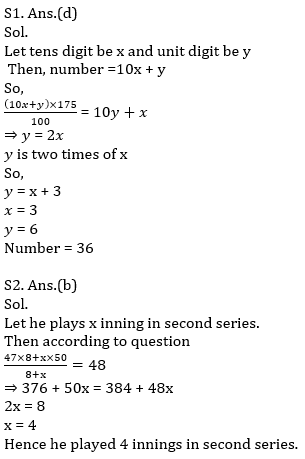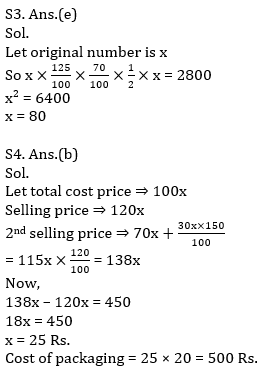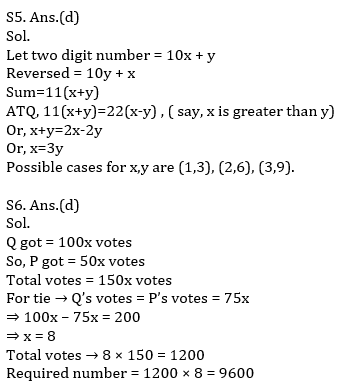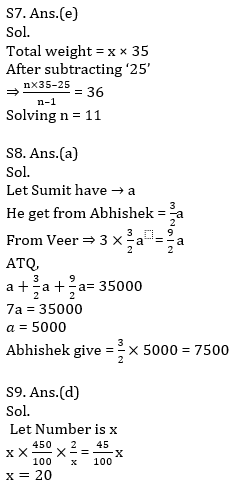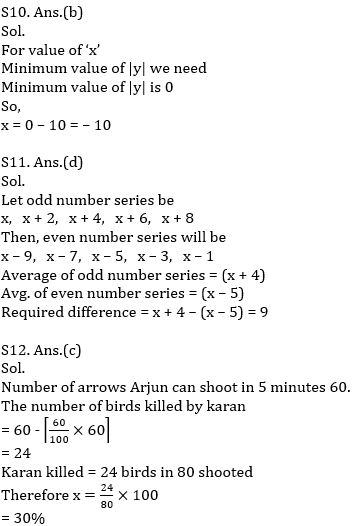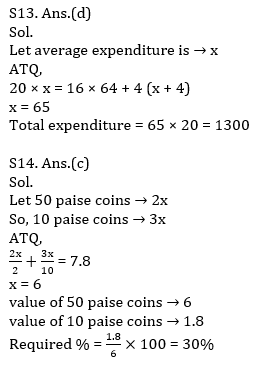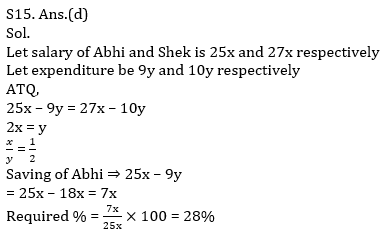#### Congratulations!